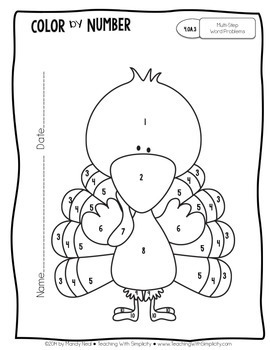# November Color By Number for 4th Grade MathSubject
Resource Type
Format
PDF (9 MB|31 pages)
Standards
\$3.25
\$3.25

#### Also included in

1. The 4th Grade Color by Number Math Bundle includes all 4th grade color by number printables. The monthly color by numbers provides students with opportunities to review skills previously learned and is aligned specifically to each grade level. Students complete a math problem, record the answer, a
\$14.63
\$29.25
Save \$14.62

### Description

The monthly color by numbers provide students with opportunities to review skills previously learned and is aligned specifically to each grade level.

Students complete a math problem, record the answer, and then color its corresponding part within the picture.

These are great for morning work, early finishers, and/or homework, while providing students with a variety.

The November Color by Number for 4th Grade covers the following standards:

4.OA.1 Multiplication Equations as Comparisons
4.OA.2 Multiplicative Comparison Word Problems
4.OA.3 Multi-Step Word Problems
4.OA.4 Prime & Composite Multiples & Factors
4.OA.5 Patterns
4.NBT.1 Place Value
4.NBT.2 Compare Numbers
4.NBT.3 Round Numbers
4.NBT.4 Add & Subtract Whole Numbers
4.NBT.5 Multiplication

Total Pages
31 pages
Included
Teaching Duration
N/A
Report this Resource to TpT
Reported resources will be reviewed by our team. Report this resource to let us know if this resource violates TpT’s content guidelines.

### Standards

to see state-specific standards (only available in the US).
Generate a number or shape pattern that follows a given rule. Identify apparent features of the pattern that were not explicit in the rule itself. For example, given the rule “Add 3” and the starting number 1, generate terms in the resulting sequence and observe that the terms appear to alternate between odd and even numbers. Explain informally why the numbers will continue to alternate in this way.
Find all factor pairs for a whole number in the range 1-100. Recognize that a whole number is a multiple of each of its factors. Determine whether a given whole number in the range 1-100 is a multiple of a given one-digit number. Determine whether a given whole number in the range 1-100 is prime or composite.
Solve multistep word problems posed with whole numbers and having whole-number answers using the four operations, including problems in which remainders must be interpreted. Represent these problems using equations with a letter standing for the unknown quantity. Assess the reasonableness of answers using mental computation and estimation strategies including rounding.
Multiply or divide to solve word problems involving multiplicative comparison, e.g., by using drawings and equations with a symbol for the unknown number to represent the problem, distinguishing multiplicative comparison from additive comparison.
Interpret a multiplication equation as a comparison, e.g., interpret 35 = 5 × 7 as a statement that 35 is 5 times as many as 7 and 7 times as many as 5. Represent verbal statements of multiplicative comparisons as multiplication equations.